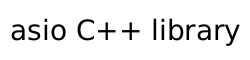The `async_read_until` function is a composed asynchronous operation that reads data into a dynamic buffer sequence, or into a streambuf, until it contains a delimiter, matches a regular expression, or a function object indicates a match.

Start an asynchronous operation to read data into a dynamic buffer sequence until it contains a specified delimiter.

```template<
typename DynamicBuffer_v1,
DynamicBuffer_v1 && buffers,
char delim,
typename constraint< is_dynamic_buffer_v1< typename decay< DynamicBuffer_v1 >::type >::value >::type  = 0,
typename constraint< !is_dynamic_buffer_v2< typename decay< DynamicBuffer_v1 >::type >::value >::type  = 0);
» more...

template<
typename DynamicBuffer_v1,
DynamicBuffer_v1 && buffers,
string_view delim,
typename constraint< is_dynamic_buffer_v1< typename decay< DynamicBuffer_v1 >::type >::value >::type  = 0,
typename constraint< !is_dynamic_buffer_v2< typename decay< DynamicBuffer_v1 >::type >::value >::type  = 0);
» more...
```

Start an asynchronous operation to read data into a dynamic buffer sequence until some part of its data matches a regular expression.

```template<
typename DynamicBuffer_v1,
DynamicBuffer_v1 && buffers,
const boost::regex & expr,
typename constraint< is_dynamic_buffer_v1< typename decay< DynamicBuffer_v1 >::type >::value >::type  = 0,
typename constraint< !is_dynamic_buffer_v2< typename decay< DynamicBuffer_v1 >::type >::value >::type  = 0);
» more...
```

Start an asynchronous operation to read data into a dynamic buffer sequence until a function object indicates a match.

```template<
typename DynamicBuffer_v1,
typename MatchCondition,
DynamicBuffer_v1 && buffers,
MatchCondition match_condition,
typename constraint< is_match_condition< MatchCondition >::value >::type  = 0,
typename constraint< is_dynamic_buffer_v1< typename decay< DynamicBuffer_v1 >::type >::value >::type  = 0,
typename constraint< !is_dynamic_buffer_v2< typename decay< DynamicBuffer_v1 >::type >::value >::type  = 0);
» more...
```

Start an asynchronous operation to read data into a streambuf until it contains a specified delimiter.

```template<
typename Allocator,
asio::basic_streambuf< Allocator > & b,
char delim,
» more...

template<
typename Allocator,
asio::basic_streambuf< Allocator > & b,
string_view delim,
» more...
```

Start an asynchronous operation to read data into a streambuf until some part of its data matches a regular expression.

```template<
typename Allocator,
asio::basic_streambuf< Allocator > & b,
const boost::regex & expr,
» more...
```

Start an asynchronous operation to read data into a streambuf until a function object indicates a match.

```template<
typename Allocator,
typename MatchCondition,
asio::basic_streambuf< Allocator > & b,
MatchCondition match_condition,
typename constraint< is_match_condition< MatchCondition >::value >::type  = 0);
» more...
```

Start an asynchronous operation to read data into a dynamic buffer sequence until it contains a specified delimiter.

```template<
typename DynamicBuffer_v2,
DynamicBuffer_v2 buffers,
char delim,
typename constraint< is_dynamic_buffer_v2< DynamicBuffer_v2 >::value >::type  = 0);
» more...

template<
typename DynamicBuffer_v2,
DynamicBuffer_v2 buffers,
string_view delim,
typename constraint< is_dynamic_buffer_v2< DynamicBuffer_v2 >::value >::type  = 0);
» more...
```

Start an asynchronous operation to read data into a dynamic buffer sequence until some part of its data matches a regular expression.

```template<
typename DynamicBuffer_v2,
DynamicBuffer_v2 buffers,
const boost::regex & expr,
typename constraint< is_dynamic_buffer_v2< DynamicBuffer_v2 >::value >::type  = 0);
» more...
```

Start an asynchronous operation to read data into a dynamic buffer sequence until a function object indicates a match.

```template<
typename DynamicBuffer_v2,
typename MatchCondition,
DynamicBuffer_v2 buffers,
MatchCondition match_condition,
Header: `asio/read_until.hpp`
Convenience header: `asio.hpp`